# 世界杯来了，让 Towhee 带你多语言「以文搜球」！

• 2022-11-26
北京
• 本文字数：4008 字

阅读完需：约 13 分钟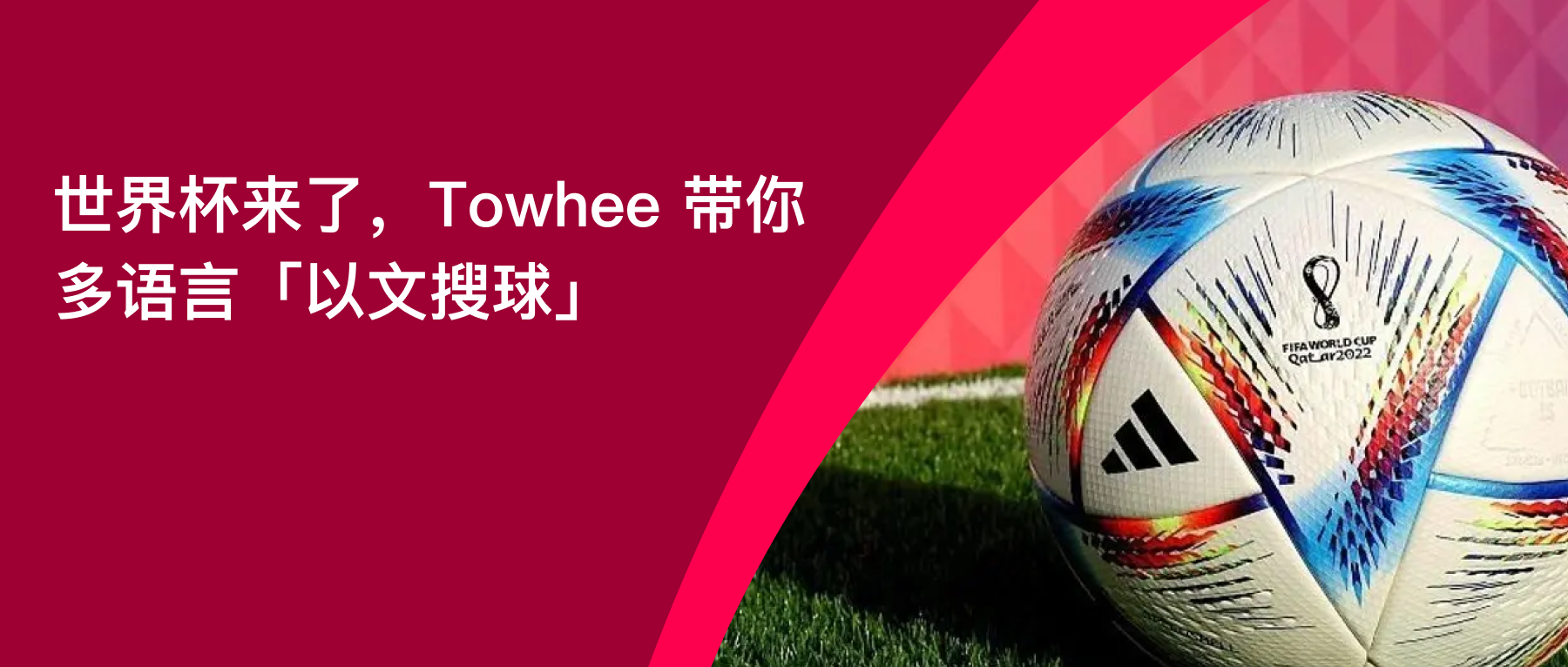「以文搜球」是跨模态图文检索的一部分，如今最热门的跨模态图文检索模型莫过于 CLIP，关于模型的原理详解和相关使用教程，推荐参考从零到一，教你搭建从零到一，教你搭建「以文搜图」搜索服务系列文章。

## 中文版搜球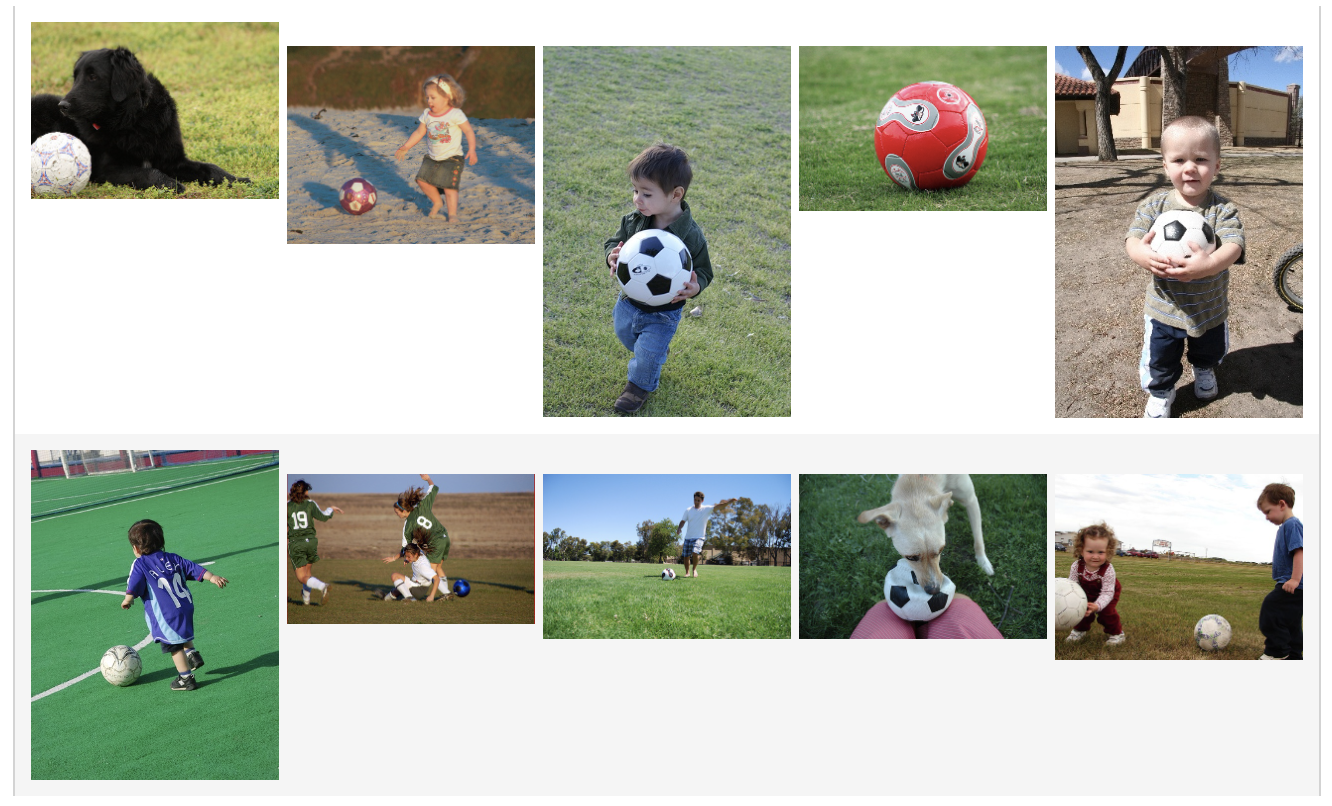• 数据：10 张图片来自图像数据集 ImageNet 中的“soccer_ball”类别，包括了足球相关的图片。

• 特征提取image_text_embedding.taiyi 能够将中文或图片转换成向量，使用同一个模型生成的向量拥有同样的特征空间。

• 向量数据库：这里搭建的「以文搜图」系统使用了 Milvus 实现向量存储与检索，包括匹配对应的图片路径。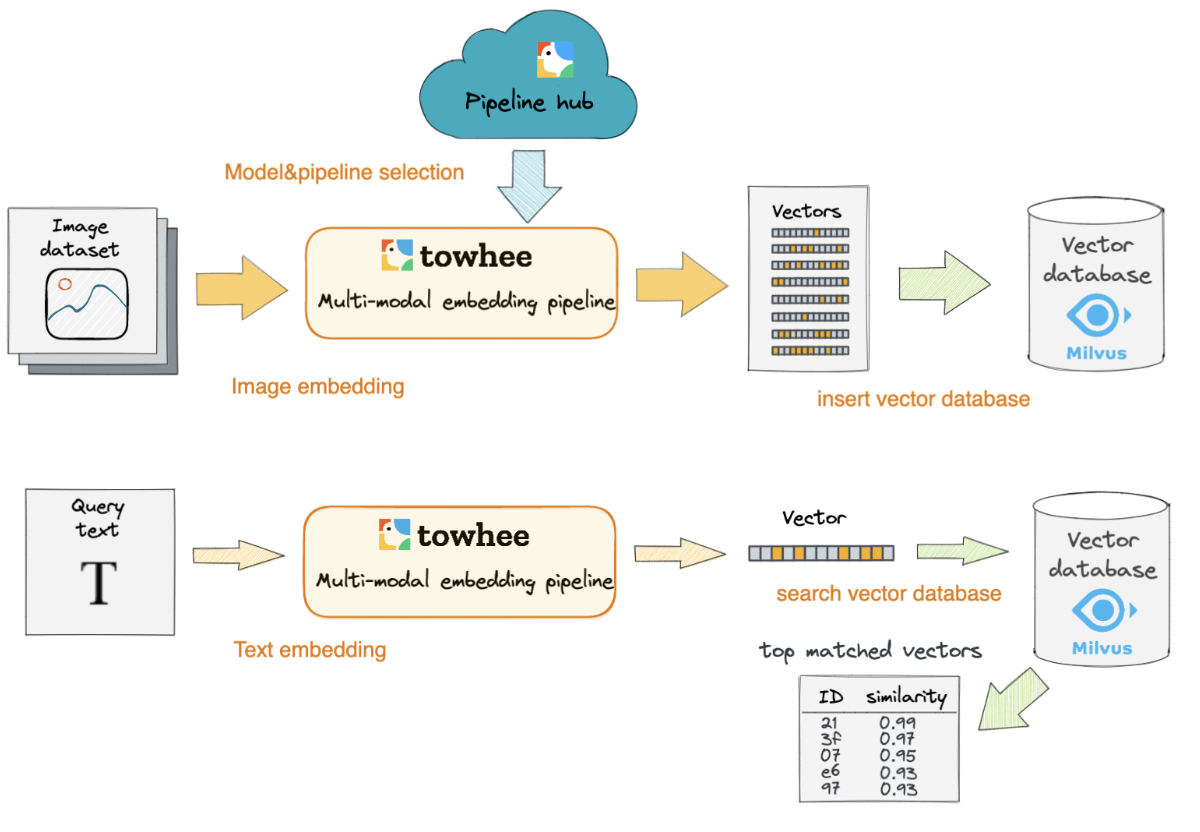### 1. 准备工作

python -m pip install towhee pymilvus

• 集合名：唯一且不重复，用于指定集合

• 数据表格：

• id：主键，由系统自动生成唯一且不重复的整数（无需插入）

• embedding：图片向量，由 512 维浮点数组成

• path：图片路径，由字段组成

• 索引：基于 embedding 列数据创建 IVF_FLAT 索引（参数 "nlist":2048），同时选择 IP 内积衡量向量之间的相似度（距离越大表示越相似）

from pymilvus import connections, FieldSchema, CollectionSchema, DataType, Collection, utilityHOST = 'localhost'PORT = '19530'COLLECTION_NAME = 'text_image_search'INDEX_TYPE = 'IVF_FLAT'METRIC_TYPE = 'IP'DIM = 512TOPK = 3def create_milvus(exist_ok=False):    try:        connections.connect(host=HOST, port=PORT)    except Exception:        raise RunTimeError(f'Fail to connect Milvus with {HOST}:{PORT}')    if utility.has_collection:        collection = Collection(COLLECTION_NAME)        if exist_ok:            print(f'Using existed collection: {COLLECTION_NAME}.')            return collection        else:            print('Deleting previous collection...')            collection.drop()    # Create collection    print('Creating collection...')    fields = [        FieldSchema(name='id', dtype=DataType.INT64, description='embedding ids', is_primary=True, auto_id=True),        FieldSchema(name='embedding', dtype=DataType.FLOAT_VECTOR, description='image embeddings', dim=DIM),        FieldSchema(name='path', dtype=DataType.VARCHAR, description='image path', max_length=500)        ]    schema = CollectionSchema(fields=fields, description='text image search')    collection = Collection(name=COLLECTION_NAME, schema=schema)    # Create index    print('Creating index...')    index_params = {        'metric_type': METRIC_TYPE,        'index_type': INDEX_TYPE,        'params':{"nlist":2048}    }    collection.create_index(field_name='embedding', index_params=index_params)    print(f'Milvus collection is ready: {COLLECTION_NAME} ({INDEX_TYPE}, {METRIC_TYPE}).')    return collection    collection = create_collection()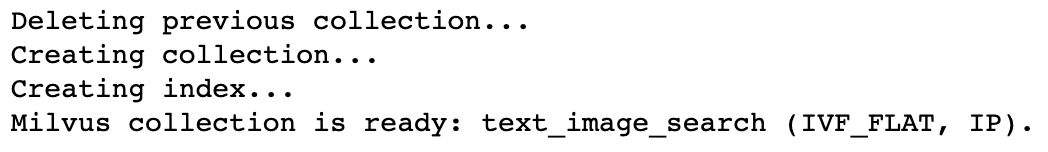### 2. 插入数据

1. 根据图片路径读取并解码图片

2. 利用预训练模型生成图片向量

3. 将向量与对应的图片路径插入事先准备好的 Milvus 集合

import towhee# Insertinsert = (    towhee.glob['path']('path/to/soccer_ball/*.JPEG')          .image_decode['path', 'image']()          .image_text_embedding.taiyi['image', 'vec'](                   model_name='taiyi-clip-roberta-102m-chinese',                   modality='image')          .ann_insert.milvus[('vec', 'path'), 'milvus_res'](                   uri=f'tcp://{HOST}:{PORT}/{COLLECTION_NAME}')#           .select['path', 'image', 'milvus_res']()#           .show())print(f'Total vectors in collection: {collection.num_entities}')

### 3. 检索测试

import towheequery = (    towhee.dc['text'](['输入查询语句'])          .image_text_embedding.taiyi['text', 'vec'](                   model_name='taiyi-clip-roberta-102m-chinese',                   modality='text')          .ann_search.milvus['vec', 'milvus_res'](                   uri=f'tcp://{HOST}:{PORT}/{COLLECTION_NAME}',                   metric_type=METRIC_TYPE,                   limit=TOPK,                   output_fields=['path'])          .flatten('milvus_res')          .runas_op['milvus_res', ('image_path', 'score')](lambda x: (x.path, x.score))          .image_decode['image_path', 'image']()          .select['text', 'image', 'score']()          .show())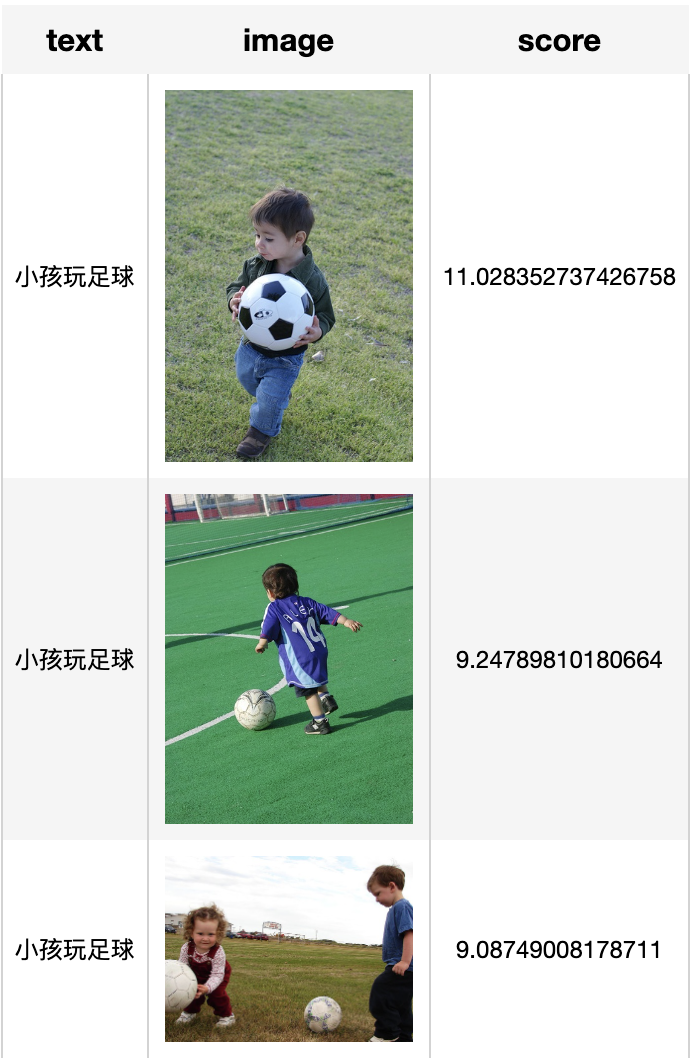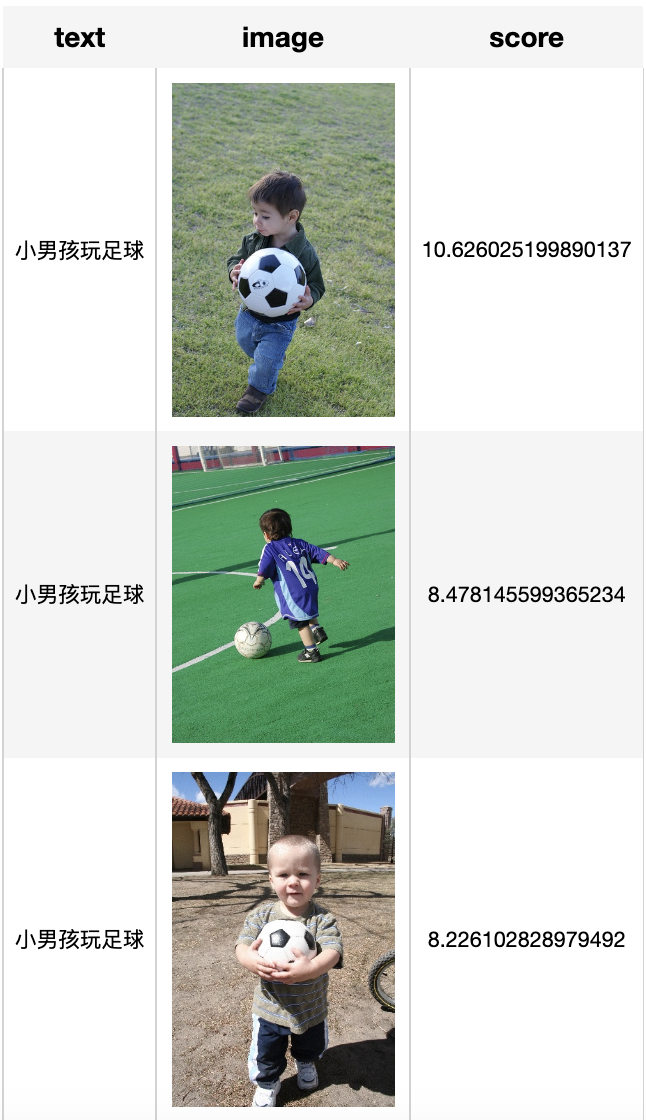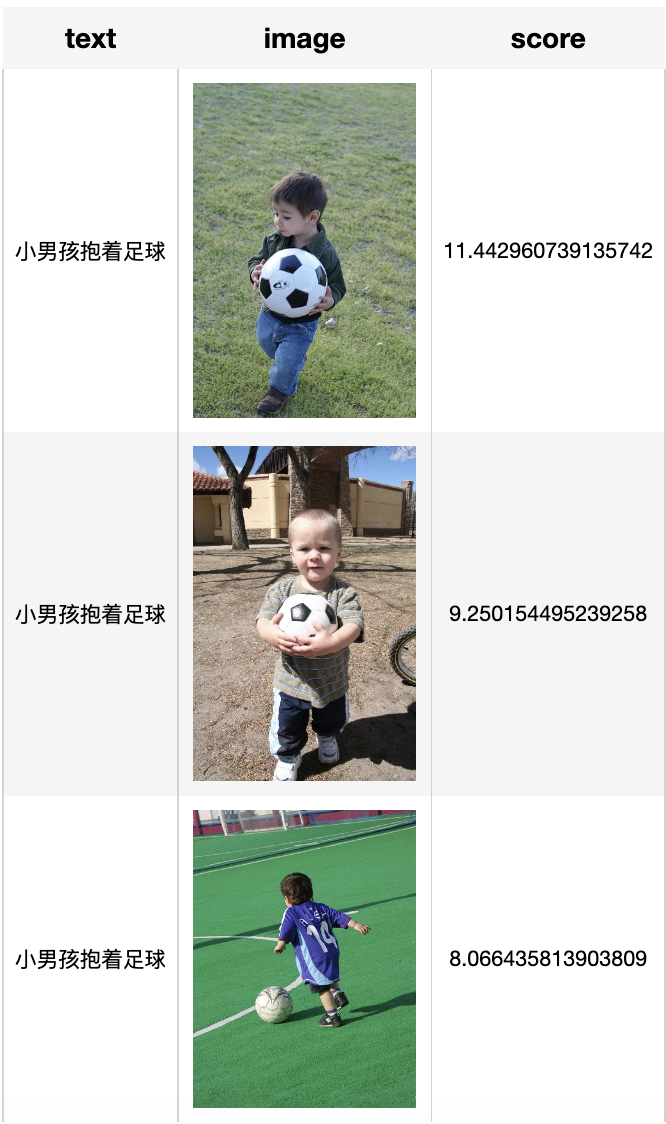## 多语言版搜球

• 自定义算子：

from towhee.models.clip import create_modelfrom torchvision import transformsfrom PIL import Imageimport numpy()model = create_model('clip_vit_b32', pretrained=True, device='cpu')def encode_text(x):    features = model.encode_text(x, multilingual=True).squeeze(0).detach().cpu().numpy()    return featuresdef encode_image(x):    tfms = transforms.Compose([        transforms.Resize(224, interpolation=transforms.InterpolationMode.BICUBIC),        transforms.CenterCrop(224),        transforms.ToTensor(),        transforms.Normalize(           (0.48145466, 0.4578275, 0.40821073), (0.26862954, 0.26130258, 0.27577711))        ])    img = Image.open(x)    x = tfms(img).unsqueeze(0)    features = model.encode_image(x).squeeze(0).detach().cpu().numpy()    return features

• 插入数据：

import towhee# Insertinsert = (    towhee.glob['path']('path/to/soccer_ball/*.JPEG')          .runas_op['path', 'vec'](func=encode_image)          .ann_insert.milvus[('vec', 'path'), 'milvus_res'](uri=f'tcp://{HOST}:{PORT}/{COLLECTION_NAME}')          .select['path', 'milvus_res']()          .show())print(f'Total vectors in collection: {collection.num_entities}')

• 文本查询：

import towheequery = (    towhee.dc['text'](['输入查询语句'])          .runas_op['text', 'vec'](func=encode_text)          .ann_search.milvus['vec', 'milvus_res'](                   uri=f'tcp://{HOST}:{PORT}/{COLLECTION_NAME}',                   metric_type=METRIC_TYPE,                   limit=TOPK,                   output_fields=['path'])          .flatten('milvus_res')          .runas_op['milvus_res', ('image_path', 'score')](lambda x: (x.path, x.score))          .image_decode['image_path', 'image']()          .select['text', 'image', 'score']()          .show())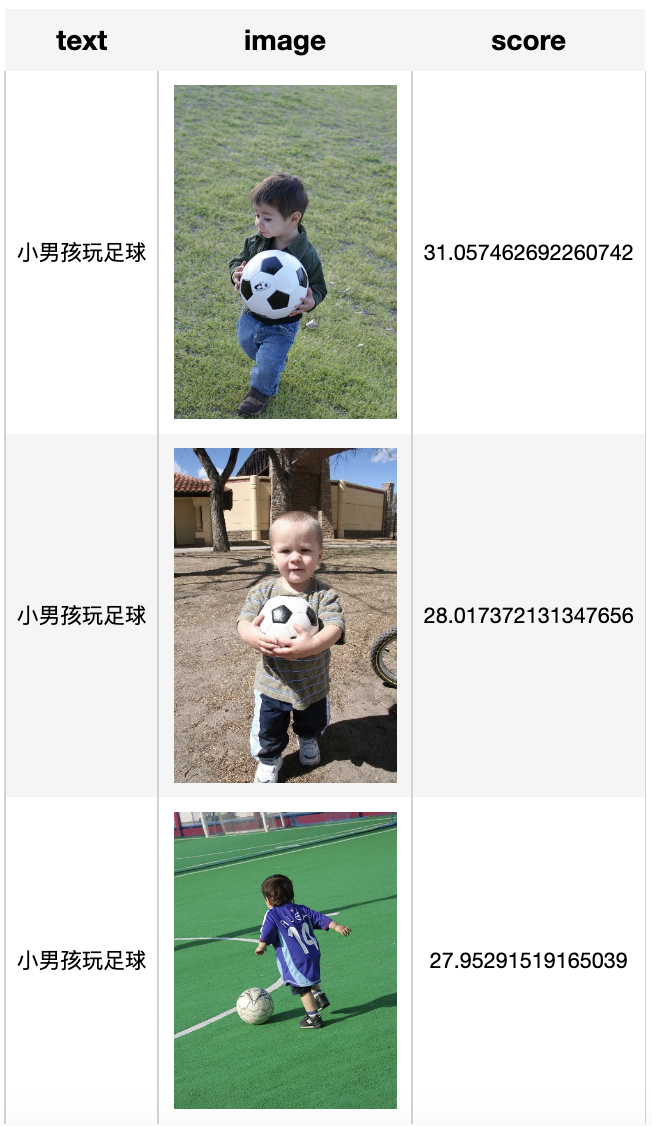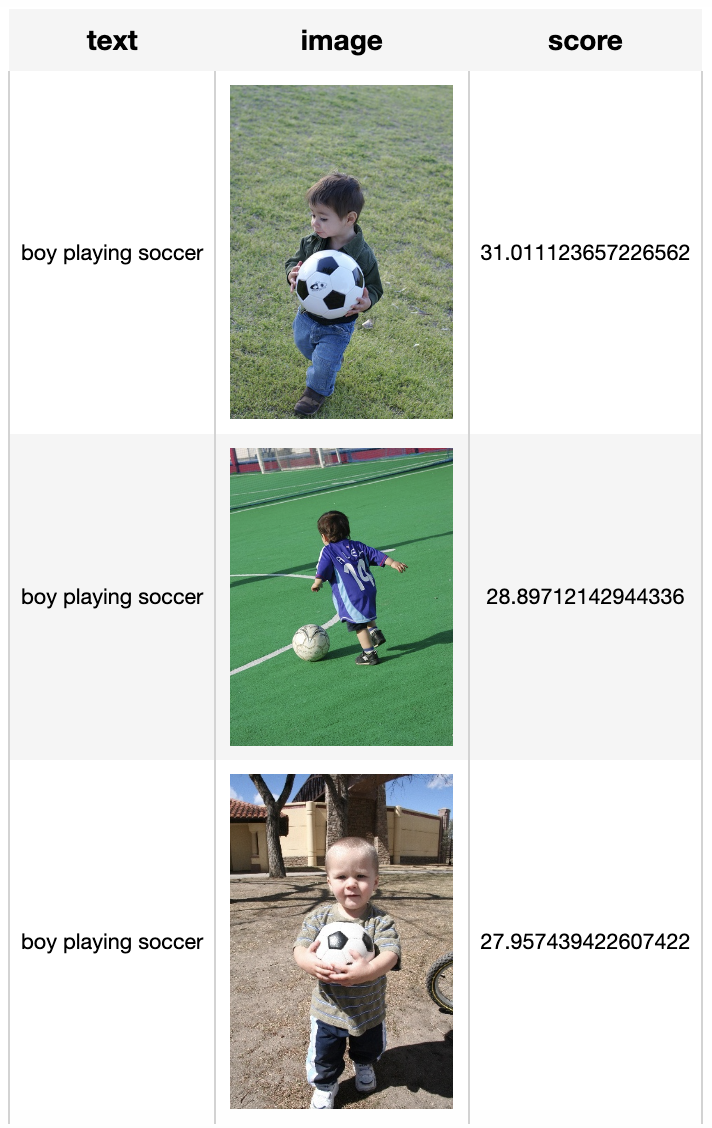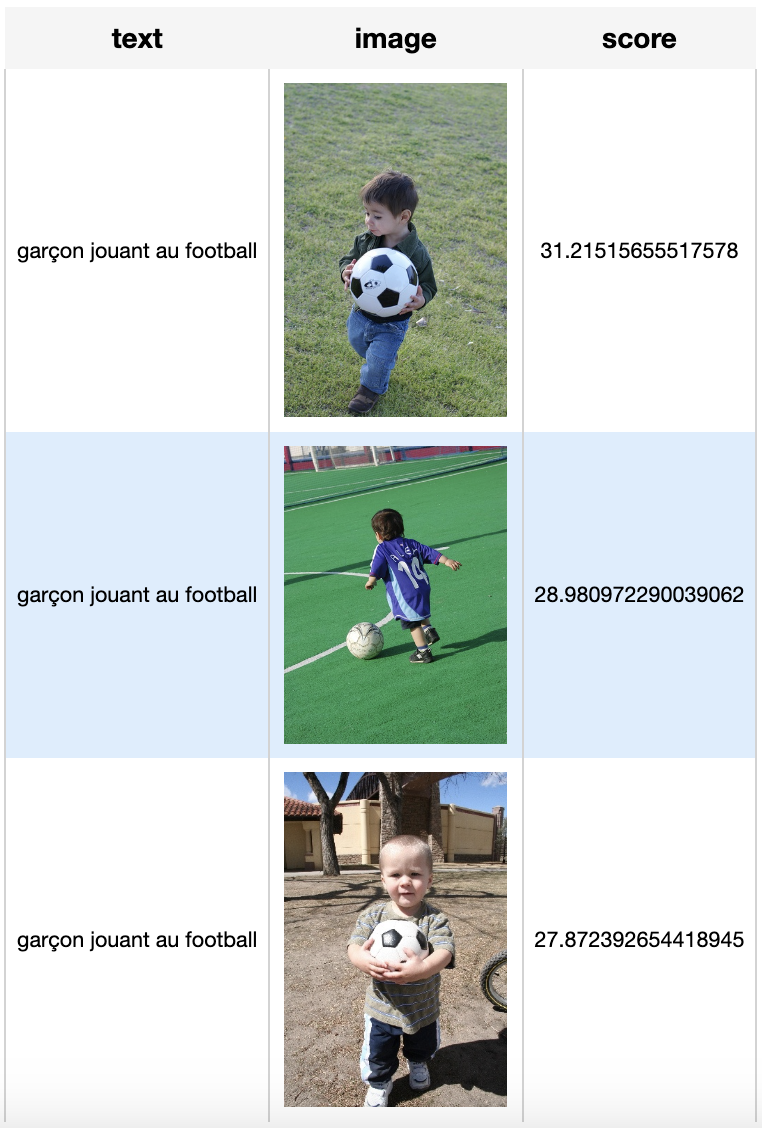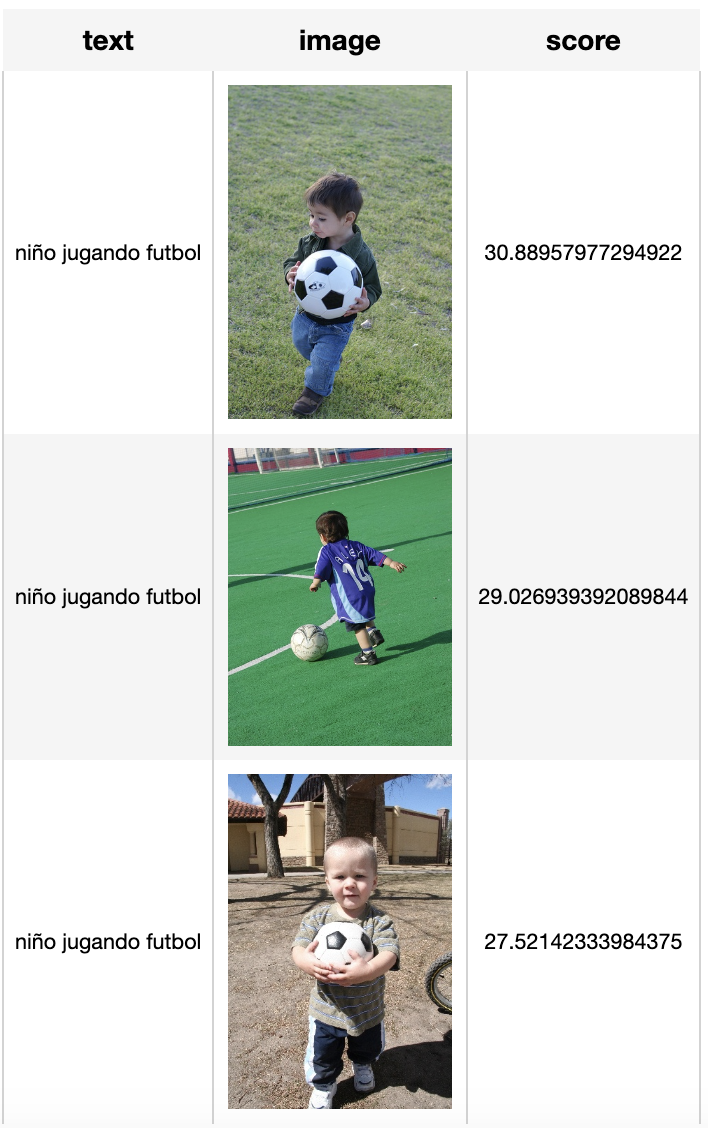### Zilliz

Reinvent data science 2021-10-09 加入

## 评论